## A nonconducting sphere of radius 10 cm is charged uniformly with a density of 100 nC/m3. What is the magnitude of the potential difference b

Question

A nonconducting sphere of radius 10 cm is charged uniformly with a density of 100 nC/m3. What is the magnitude of the potential difference between the center and a point 4.0 cm away?

in progress 0
1 month 2021-08-14T06:58:16+00:00 1 Answers 1 views 0

1. Explanation:

The given data is as follows.

Radius of the sphere (R) = 10 cm = 0.01 m   (as 1 m = 100 cm)

Distance from the center (r) = 4 cm = 0.04 m

Charge density (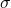) = 100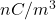=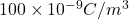(As 1 nm =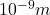)

As the relation between charge and potential difference is as follows.

Q =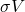=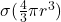=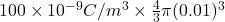=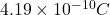Expression for electric field is as follows.

E(r) =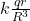Electric potential, V(r) =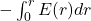=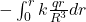=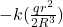=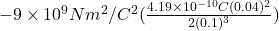= -3 V

Thus, we can conclude that the magnitude of the potential difference between the center and a point 4.0 cm away is -3 V.